Courses

# Test: Optical Isomerism (Isomerism)

## 20 Questions MCQ Test Chemistry Class 11 | Test: Optical Isomerism (Isomerism)

Description
This mock test of Test: Optical Isomerism (Isomerism) for Class 11 helps you for every Class 11 entrance exam. This contains 20 Multiple Choice Questions for Class 11 Test: Optical Isomerism (Isomerism) (mcq) to study with solutions a complete question bank. The solved questions answers in this Test: Optical Isomerism (Isomerism) quiz give you a good mix of easy questions and tough questions. Class 11 students definitely take this Test: Optical Isomerism (Isomerism) exercise for a better result in the exam. You can find other Test: Optical Isomerism (Isomerism) extra questions, long questions & short questions for Class 11 on EduRev as well by searching above.
QUESTION: 1

Solution:
QUESTION: 2

### Find the number of stereoisomers for CH3 – CHOH – CH = CH – CH3.

Solution:

The number of stereoisomers for CH3 – CHOH – CH = CH – CH3 is four. This is calculated by the formula 2n+1.

QUESTION: 3

### A hydrocarbon X is optically. X upon hydrogenation gives an optically inactive alkane Y. Which of the following pair of compounds can be X and Y respectively?

Solution:

The correct answer is Option B.
The optically active hydrocarbon X is 3-methyl-1-pentene CH2=CH−CH(CH3)CH2CH3​. On catalytic hydrogenation, it forms 3-methyl pentane CH3CH2CH(CH3)CH2CH3, which is optically inactive.

QUESTION: 4

What is wrong about enantiomers of 2-chloropropanoic acid?

Solution:

The correct answer is option D.
Pair of enantiomers react differently with pure enantiomers of other compounds.

QUESTION: 5

How many diffrent stereoisomers exist for the compound below ?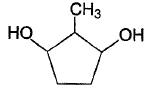Solution:

If you name the C attached to Me group as C1 and naming the rest clockwise, you observe that at C1 and C2 there are chiral centers and C5 and C2 are symmetrical. So the two chiral centers produce 2×2=4 optically active(and distinguishable) compounds. Hence B is the answer.

QUESTION: 6

How many total cyclic isomers are possible for C5H10 ?

Solution:

The correct answer is Option B.

5 isomers are possible. C5H10(CnH2n). Molecules having the CnH2n formulas are most likely to be cyclic alkanes. The five isomers are: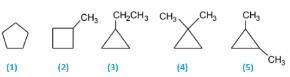(1)   Cyclopentane
(2) 1-Methylcyclobutane
(3) 1-Ethylcyclopropane
(4) 1,1-Dimethylcyclopropane
(5) 1,2-Dimethylcyclopropane

QUESTION: 7

How the following pair of isomers cannot be distinguished ?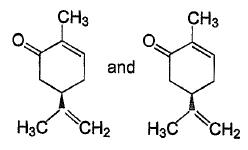Solution:
QUESTION: 8

Which compound below exhibit stereolsomerism?

Solution: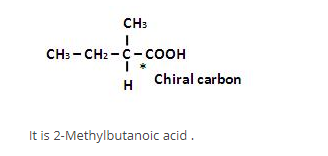QUESTION: 9

Consider all possible isomeric ketones, including stereoisomers of Molecular weight 100. All these isomers are independently reacted with NaBH4
(Note stereoisomers are also reacted separately). The total number of ketones that give a racemic product(s) is/are

Solution:

The general formula of isomeric ketone having molecular mass 100 is C6​H12​O [6×12+12×1+16].
The possible structure will be :
CH3​−CH2​−CH2​−C∣∣O−CH3​
CH3​CH2​CH2​−C∣∣O−CH2​CH3​
CH3​−CH3​∣CH​−CH2​−C∣∣O−CH3
CH3​−CH2​−C∣HCH3​−C∣∣O−CH3
CH3​−C∣HCH3​−C∣∣O−CH2​−CH3​
CH3​−CH3​∣C​​∣​CH3​​−C∣∣O−CH3
The number of ketones that gives racemic mixture with NaBH4 is 5 as the ketone with chiral center will give diastereoisomer with NaBH4​

*Multiple options can be correct
QUESTION: 10

Direction (Q. Nos. 10-14) This section contains 5 multiple choice questions. Each question has four
choices (a), (b), (c) and (d), out of which ONE or  MORE THANT ONE  is correct.

Q.

Consider the followring molecule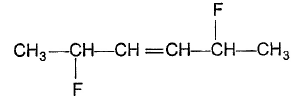The correct statement concerning above molecule is/are

Solution:
*Multiple options can be correct
QUESTION: 11

Consider  the following free radicl chlorination reaction.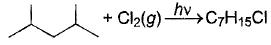The correct statement about product(s) is/are

Solution:

There are 4 final products, they are as follow:-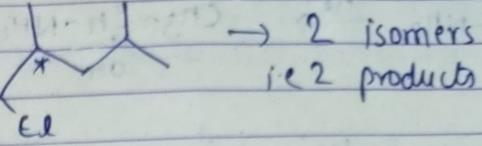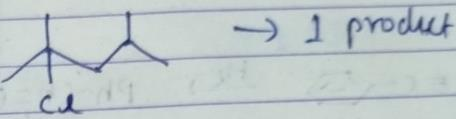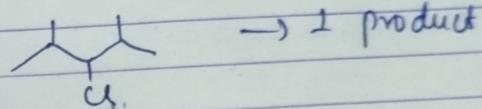*Multiple options can be correct
QUESTION: 12

The correct statement(s) regarding Isomers of a compound with general name trichlorocyclobutane is/are

Solution:
*Multiple options can be correct
QUESTION: 13

The correct statement (s) about the compound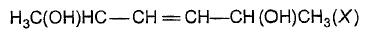is/are

[IIT JEE 2009]

Solution:

The correct options are A & D
Since a carbon-carbon double bond is present, the molecule can have either cis isomer or trans isomer.
In cis isomer (which is symmetrical), the number of enantiomers is
2(n−1)=2(2−1)=2
The number of meso compounds possible is 2(n/2)−1=2(2/2)−1=1
Hence, the total number of optical isomers possible is 2+1=3.
Trans isomer is also symmetrical.
Hence, the total number of optical isomers possible for trans isomers is also 3.
Hence, the total number of stereoisomers possible for X is 3+3=6.
Hence, option A is correct and option B is incorrect.
If the stereochemistry about the double bond in X is trans, the number of enantiomers possible for X is 4.
Thus, the option C is incorrect.
If the stereochemistry about the double bond in X is cis, the number of enantiomers possible for X is 2.
Thus the option D is correct.

*Multiple options can be correct
QUESTION: 14

The correct statement(s) about the compound given below is/are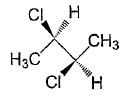Solution:

The correct answer is option A & D
The given image is Self-Explanatory.
We know that following are the cases when a compound is Optically Inactive:
1. When it does not have a chiral Carbon Atom eg.: Methyl bromide
2. When it has a Plane or Axis of Symmetry eg.: Tartaric Acid (these compounds are called as Meso Compounds)
3. When it forms a Racemic Mixture eg.: Equimolar mixture of d-Lactic Acid and l-Lactic Acid.
The given compound in the question possesses an Axis of Symmetry (shown in the attached image). Hence, it is Optically Inactive.

QUESTION: 15

Direction (Q. Nos. 15-20) This section contains 2 paragraph, wach describing  theory, experiments, data etc. three Questions related to paragraph have been  given.Each question have only one correct answer among the four given  ptions  (a),(b),(c),(d)

Passage I

Five alkenes, structural isomers, based on general formula CnH2n, each on hydrogenation, gives the same, smallest optically active alkane.

Q.

How many of the above alkene(s) is/are capable of showing geometrical isomerism?

Solution:
QUESTION: 16

Passage I

Five alkenes, structural isomers, based on general formula CnH2n, each on hydrogenation, gives the same, smallest optically active alkane.

Q.

if for the above five alkenes, their stereoisomers are also included, the total number of isomers would become

Solution:
QUESTION: 17

Passage I

Five alkenes, structural isomers, based on general formula CnH2n, each on hydrogenation, gives the same, smallest optically active alkane.

Q.

how many isomers in the previous qre capable of rotating plane polarised ?

Solution:
QUESTION: 18

Passage II
Organic compound with molecular formula C3H6O has one degree of unsaturation. One degree of unsaturation can be present in the form of a pi-bond or a ring structure. Pi-bond can be formed between carbon atoms or between carbon and oxygen atom.

Q.

How many isomers exist for this compound that have a ring structure?

Solution: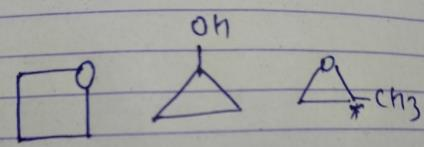Last one has a chiral centre, So it will have 2 isomers.
So, we have 4 isomers in all.

QUESTION: 19

Passage II
Organic compound with molecular formula C3H6O has one degree of unsaturation. One degree of unsaturation can be present in the form of a pi-bond or a ring structure. Pi-bond can be formed between carbon atoms or between carbon and oxygen atom.

Q.

If only carbonyl isomers (aldehyde and ketone) are considered, how many corresponding enol isomers would be possible?

Solution:
QUESTION: 20

Passage II
Organic compound with molecular formula C3H6O has one degree of unsaturation. One degree of unsaturation can be present in the form of a pi-bond or a ring structure. Pi-bond can be formed between carbon atoms or between carbon and oxygen atom.

Q.

How many isomers of this compound exis that have no stereoisomer ?

Solution: